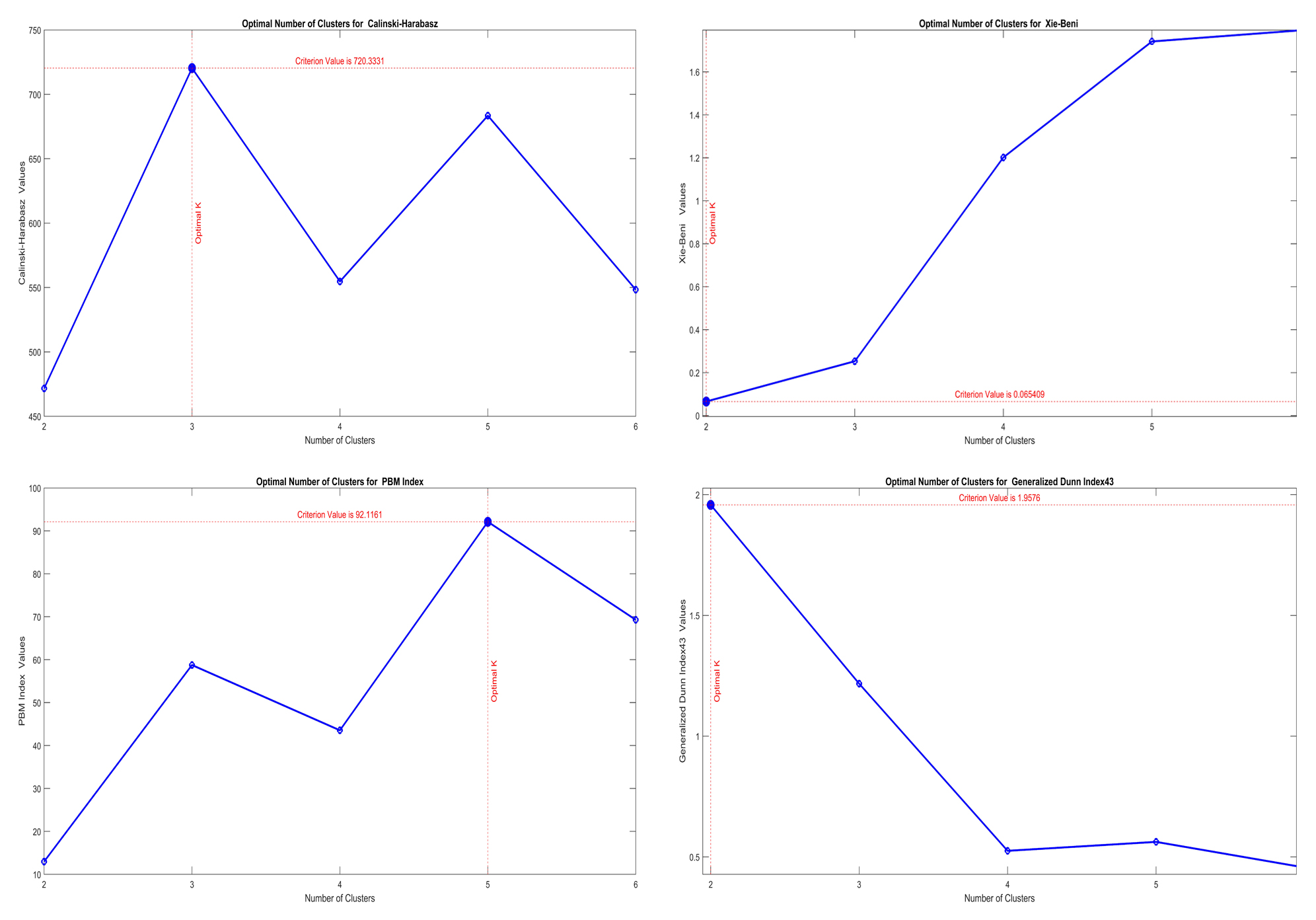## Auto-CVI-Tool , An Automatic Cluster Validity Index Toolbox

Version 1.0.0 (132 KB) by
Automatic toolbox for Cluster Validity Indexes (CVI) to determine the number of clusters automatically

Updated 6 Oct 2022

From GitHub

# `Auto-CVI-Tool`

An Automatic Toolbox for Cluster Validity Indexes (CVI)

A cluster analysis involves identifying the optimal number and natural division of clusters through automatic clustering. A cluster validity index (CVI) is a simple technique for estimating the number of clusters. Several cluster solutions have been proposed in the literature in terms of intra-cluster cohesiveness and inter-cluster separation. In spite of this, it is crucial to identify the situations where these CVIs work well and their limitations. To estimate the number of clusters, this toolbox presents 28 robust CVIs. It is extremely user-friendly and does not require any coding knowledge. Without writing a single line of code, it is possible to compare 28 CVIs and visualize the results in a comparable manner. When the data is loaded, all parameters will be automatically selected by the user, or the default setting will be used, and the CVIs can be compared without any additional programming. It is important to note that one section of this paper was used in the development of this toolbox,I would appreciate a citation to both the reference article and to myself if you use any part of this toolbox.

A cluster validity index (CVI) estimates the quality of a clustering solution by defining a relationship between intracluster cohesiveness (within-group scatter) and intercluster separation (between-group scatter). `Table1` summarizes the 22 CVIs examined in this toolbox. Each CVI is identified by an acronym in the table, which is followed by an up arrow `↑` or a down arrow `↓` to indicate whether the index is maximized or minimized, respectively.

# `Table1`

no. Index Full Name & Accronym Min\Max
1 chindex Calinski-Harabasz index (ch). `↑`
2 cindex C index (cind). `↓`
3 copindex COP index (cop). `↓`
4 csindex CS index (cs). `↓`
5 cvddindex Index based on density-involved distance (cvdd). `↑`
6 cvnnindex Index based on nearest neighbors (cvnn). `↓`
7 dbindex Davies-Bouldin index (db). `↓`
8 db2index Enhanced Davies-Bouldin index (db2). `↓`
9 dbcvindex Density-based index (dbcv). `↑`
10 dunnindex Dunn index (dunn). `↑`
11 gd31index Dunn index variant 3,1 (gd31). `↑`
12 gd33index Dunn index variant 3,3 (gd33). `↑`
13 gd41index Dunn index variant 4,1 (gd41). `↑`
14 gd43index Dunn index variant 4,3 (gd43). `↑`
15 gd51index Dunn index variant 5,1 (gd51). `↑`
16 gd53index Dunn index variant 5,3 (gd53). `↑`
17 lccvindex Index based on local cores (lccv). `↑`
18 pbmindex PBM index (pbm). `↑`
19 sdbwindex S_Dbw validity index (sdbw). `↓`
20 sfindex Score Function index (sf). `↑`
21 silindex Silhouette index (sil). `↑`
22 ssddindex Index based on shapes, sizes, densities, and separation distances (ssdd). `↓`
23 svindex SV index (sv). `↑`
24 symindex Symmetry index (sym). `↑`
25 symdbindex Davies-Bouldin index based on symmetry (sdb). `↓`
26 symdunnindex Dunn index based on symmetry (sdunn). `↑`
27 wbindex WB index (wb). `↓`
28 xbindex Xie-Beni index (xb). `↓`

# `How to Use?`

There are two scripts which are named `KMeans_Evaluation.m` and `Hierarchichal_Evaluation.m`; they evalute the clustering based on `KMeans` and `Hierarchichal Clustering` resepctively.

• `KMeans_Evaluation.m` parameter settings
• `data` : data
• `load data`
• `DistanceKMeans` : Distance Type for k-means clustering `(Table2)`
• ``````DistanceKMeans = DistKMeans;
``````
• `Kmax` : Maximum Number of Clusters
• ``````Kmax = 6; % Maximum Number of Cluster
clust = zeros(size(data,1),Kmax);
for k=1:Kmax
clust(:,k) = kmeans(data,k,'distance',DistanceKMeans);
end
``````
• `CVI` : Select form `(Table1)`
• ``````%% Select CVI
CVI = Select_CVI_KMeans;
% Evaluation of the clustering solutions
eva = evalcvi(clust,CVI, data);
``````

# `Table2`

No. Distance
2 sqeuclidean
3 cityblock
4 hamming
5 correlation
6 cosine

You may compare multiple CVIs simultaneously by executing the following code:

``````CVIs = Select_Multiple_CVI_KMeans;
Multiple_Result = Do_Multiple(CVIs,clust,data);
``````

Also it's possible to visualize the reuslt automatically.

• `Hierarchichal_Evaluation.m` parameter settings
• `data` : data
• `load data`
• `HierarchichalMethod` : Method for Hierarchical Cluster Tree `(Table3)`
• `````` Z = linkage(data, HierarchichalMethod);
``````
• `Kmax` : Maximum Number of Clusters
• ``````Kmax = 6; % Maximum Number of Cluster
for k=1:Kmax
clust(:,k) = cluster(Z, 'maxclust', k);
end
``````
• `DistanceType` : Type of pairwise distance between two sets of observations `(Table4)`
• `````` DistanceType = Distance_PDIST2;
DXX = pdist2(data,data,DistanceType);
``````
• `CVI` : Select form `(Table1)`
• `````` CVI = Select_CVI_Hierarchichal;
eva = evalcvi(clust,CVI, DXX);
``````

If you wish to compare multiple CVIs,run following code

``````CVIs = Select_Multiple_CVI_Hierarchichal;
Multiple_Result = Do_Multiple(CVIs,clust,DXX);
``````

No. Method
2 average
3 centroid
4 complete
5 median
6 single
7 ward

# `Table4`

euclidean seuclidean
squaredeuclidean cityblock
minkowski jaccard
chebychev mahalanobis
correlation cosine
spearman hamming

# `Visualization`## Refrences

``````(1) A. José-García and W. Gómez-Flores.
A survey of cluster validity indices for automatic data clustering using differential evolution.
The Genetic and Evolutionary Computation Conference* (GECCO '21), Lille, France, 2021.
DOI: 10.1145/3449639.3459341
GitHub. Retrieved October 6, 2022.
``````

## Further Question### Cite As

##### MATLAB Release Compatibility
Created with R2022a
Compatible with R2020b to R2022b
##### Platform Compatibility
Windows macOS Linux

### Community Treasure Hunt

Find the treasures in MATLAB Central and discover how the community can help you!

Start Hunting!

#### functions

Version Published Release Notes
1.0.0

To view or report issues in this GitHub add-on, visit the GitHub Repository.
To view or report issues in this GitHub add-on, visit the GitHub Repository.